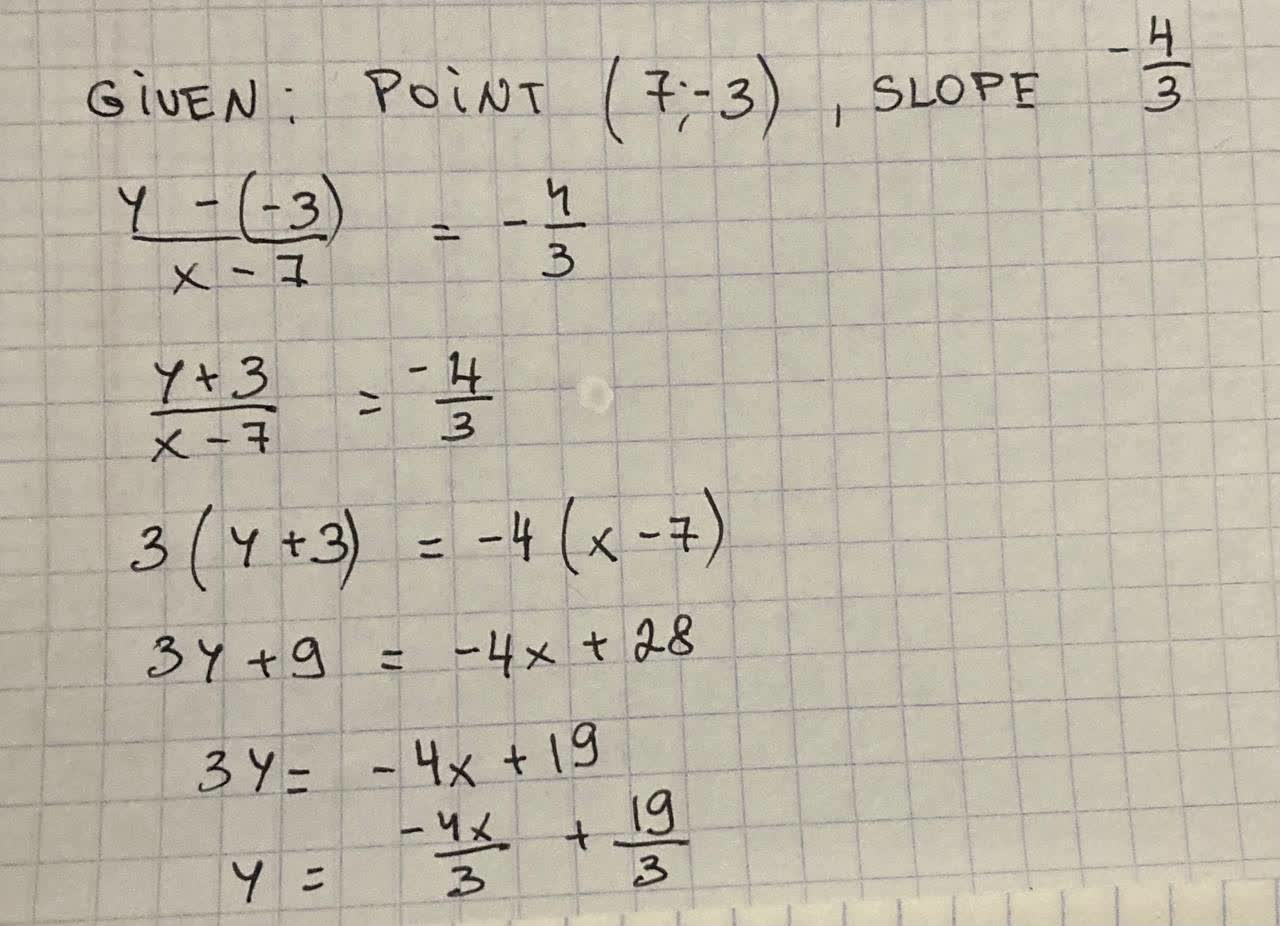# Determine the equation of the line in slope-intercept form whichRamsey 2021-08-17 Answered
Determine the equation of the line in slope-intercept form which passes through the point (7. -3) and has the slope $-\frac{4}{3}$.
You can still ask an expert for help

• Questions are typically answered in as fast as 30 minutes

Solve your problem for the price of one coffee

• Math expert for every subject
• Pay only if we can solve itfirmablogF

Explanation: